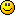#### Topic: is this an error in the "Top Bottom Price Indicator"?

hi
is this an error in the "Top Bottom Price Indicator"?
line 221
else if (slotType == SlotTypes.Close)
{
Component.CompName = "Long position closing price";
Component.DataType = IndComponentType.CloseLongPrice;
Component.CompName = "Short position closing price";
Component.DataType = IndComponentType.CloseShortPrice;
}
else if (slotType == SlotTypes.CloseFilter)
{
Component.CompName = "Close out long position";
Component.DataType = IndComponentType.ForceCloseLong;
Component.CompName = "Close out short position";
Component.DataType = IndComponentType.ForceCloseShort;

should the Component[#].DataType be switched between the two Slot types ie the IndComponentType.CloseLongPrice for the SlotTypes.CloseFilter and the IndComponentType.ForceCloseLong; for the SlotTypes.Close?  or are they correct the way they are?
also, since we are on the subject of this indicator, could someone explaine this commands?
line line 135
double dShift = IndParam.NumParam.Value * Point;
what is the function of the "Point"
and  line 145
double dTop    = double.MinValue;
double dBottom = double.MaxValue;
how to interpret, and when to use the MinValue/MaxValue?
Thanks

#### Re: is this an error in the "Top Bottom Price Indicator"?

1. Change component type:
We change the component type for the different slots because the purpose of the slot(component) is different.
- When an indicator is at "slotType == SlotTypes.Close", it represent an actual price level, where we have to close a position once the level was reached. The values of such components are prices.
The components types are:
Component.DataType = IndComponentType.CloseLongPrice; which is the price at we have to close a long position, and
Component.DataType = IndComponentType.CloseShortPrice;; price for closing a short position.
The corresponding components names are:
Component.CompName = "Long position closing price";
Component.CompName = "Short position closing price";

- When an indicator is in "Closing Logic Condition" slot, it acts like filter that has to activate position closing. Its value is a True or False (0 or 1). The components types are accordingly:
Component.DataType = IndComponentType.ForceCloseLong; and
Component.DataType = IndComponentType.ForceCloseShort;
We use the word "Force" because the interaction logic of the closing logic slots is "OR". That means that when even one satisfied logical condition is enough to "force" the closing.

2. What is function of Point in double dShift = IndParam.NumParam.Value * Point;
Point = 0.0001 for 4 digit broker and Point = 0.00001 for 5 digit broker.
User enters the Shift parameter as an integer number, let say 20.
Multiplying by Point makes the Shift proper for calculation the price.
Example: Top = 1.26356, Shift = 20
Shifted Top Price = Top + Shift * Point = 1.26356 + 0.00001 * 20 = 1.26356 + 0.00020 = 1.26376

3.   Purpose of double dTop    = double.MinValue;
This is a standard way of initialization of a variable when searching for a higher value. double.MinValue is the absolutely minimal value a double number can take.
Later on the code we have:
if (High[iBar - 1] > dTop)
dTop = High[iBar - 1];
So, when the High of previous bar is higher than the current dTop, we make dTop = Previous High.
We set dTop to double.MinValue before checking the previous High in order to be sure that it is lower than what ever a High price can be.

#### Re: is this an error in the "Top Bottom Price Indicator"?

Thank you very much for the detailed explanation, the word "FORCE" is what made me believe that the two IndComponentType were reversed, and since they do have similar functions they might have been missed in testing.
now I understand the reason.... basicaly when the condition becomes true in one of the closing filters it might not close the position unless the IndComponentType has a FORCE command in it, without it the program will keep the position open until all filters are congruent on closing the position... I guess the filters also must belong to the same group to function that way...

Thank you again